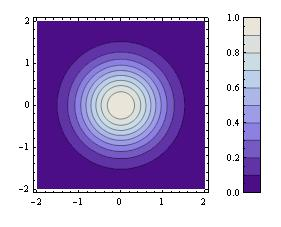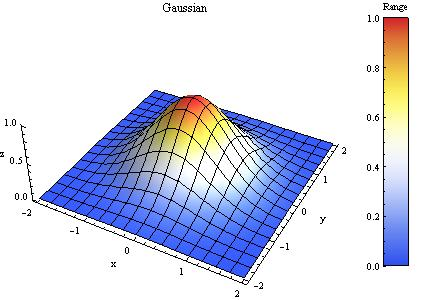## Mathematica version of colorbar

October 31st, 2007 | Categories: math software, mathematica | Tags:

Imagine that you have a function of the form z=f(x,y) and you want to plot it. The result you have in mind is something like this:The above plot was produced by Matlab and the scale on the right hand side is produced via the colorbar function. I was recently asked if it is possible to easily produce such a scale using Mathematica and after a long search through the documentation the answer was no! Just to make sure that I hadn’t missed something I made a post to comp.soft-sys.mathematica to see what the Mathematica gurus there would make of the problem.

Sure enough there is no simple alternative to colorbar in Mathematica but some helpful soul posted a piece of code that reproduced a similar effect. With a bit of work this turned out to be sufficient for the needs of the person who originally approached me and everyone was happy.

A few days later I discovered that someone called Will Robertson had been following the same thread on the newsgroup and he had used the information posted there to produce a package that allowed a Mathematica user to easily produce ‘colorbar plots’ for both DensityPlots and ContourPlots. I hacked his code so that it supported Plot3D as well and emailed it to him. Between us we tidied up the code a little and submitted it to the wolfram library for everyone to download and use for free.

There is an examples file included in the package distribution that shows how to use it in detail but to give you an idea of what you are going to get here is how to plot the functiononce you have downloaded and run the package. The command

ColorbarPlot[Exp[-(#1^2 + #2^2)] &, {-2, 2}, {-2, 2}]

produces the following plotThe above plot is a DensityPlot which is the default type but you can change it using the PlotType option:

ColorbarPlot[Exp[-(#1^2 + #2^2)] &, {-2, 2}, {-2, 2},PlotType->ContourPlot]Valid PlotTypes are DensityPlot, ContourPlot and Plot3D but this list will be expanded in future versions. Finally, here is a plot that makes use of lots of options.

f = Exp[-(#1^2 + #2^2)] &
plot = ColorbarPlot[f, {-2, 2}, {-2, 2},
PlotType -> Plot3D,
XLabel -> “x”,
YLabel -> “y”,
ZLabel -> “z”,
Title -> “Gaussian”,
CLabel -> “Range”,
Height -> 300,
Colors -> “TemperatureMap”,
Boxed -> False
]The package is available for download from here. Comments are welcomed and we hope you like it.

• UPDATE 12th Novermber 2010. Update to version 0.6
• UPDATE 22nd October 2009. Update to version 0.5
• UPDATE 5th June 2008. This package has now been updated to version 0.4
1. Maybe I missed it, but is there a way to not have the color bar limits autoscale with the data? Something along the lines in Matlab of doing:
caxis([vmin vmax])
which fixes the colorscale between vmin and vmax, regardless of the data being plotted by contour or pcolor (again, using Matlab commands).

2. Is this package at “end of life” now that Wolfram have added Plot/Bar legends in V9?